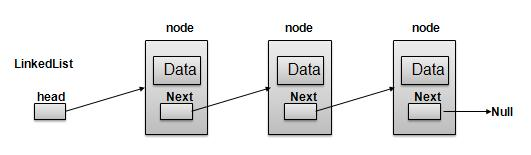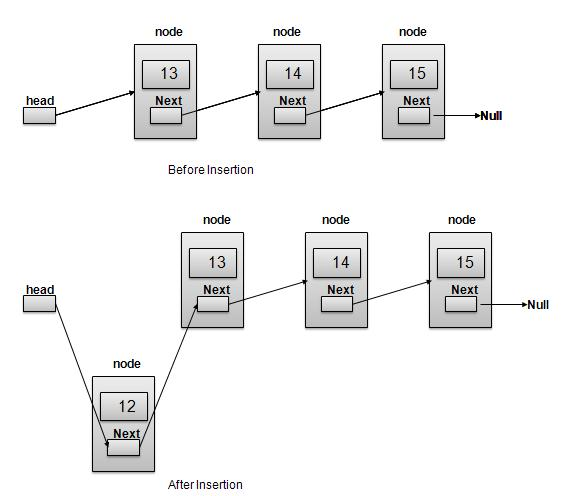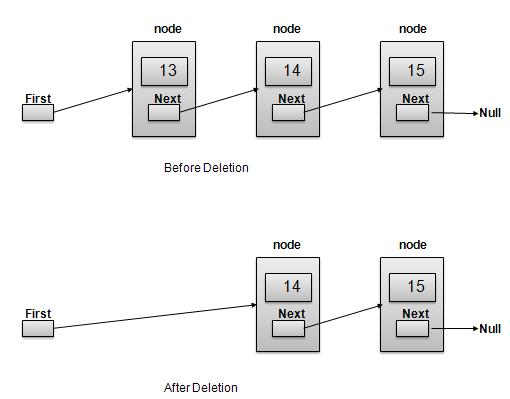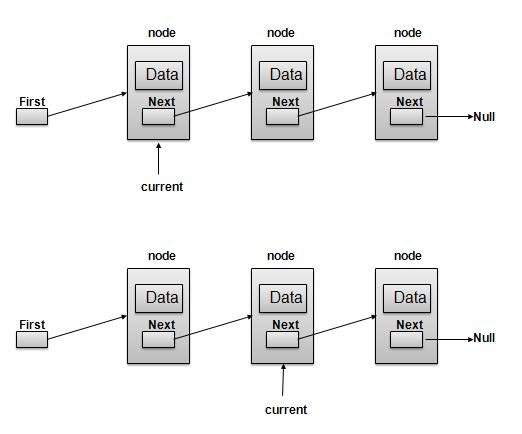# Data Structure - Linked List

A linked-list is a sequence of data structures which are connected together via links.

Linked List is a sequence of links which contains items. Each link contains a connection to another link. Linked list the second most used data structure after array. Following are important terms to understand the concepts of Linked List.As per above shown illustration, following are the important points to be considered.

• Each Link carries a data field(s) and a Link Field called next.
• Last Link carries a Link as null to mark the end of the list.

Following are the various flavours of linked list.

• Doubly Linked List − Items can be navigated forward and backward way.

• Circular Linked List − Last item contains link of the first element as next and and first element has link to last element as prev.

## Basic Operations

Following are the basic operations supported by a list.

• Insertion − add an element at the beginning of the list.

• Deletion − delete an element at the beginning of the list.

• Display − displaying complete list.

• Search − search an element using given key.

• Delete − delete an element using given key.

## Insertion Operation

Insertion is a three step process −

• Create a new Link with provided data.```//insert link at the first location
void insertFirst(int key, int data){
struct node *link = (struct node*) malloc(sizeof(struct node));

//point it to old first node

//point first to new first node
}
```

## Deletion Operation

Deletion is a two step process −```//delete first item
struct node* deleteFirst(){

//mark next to first link as first

}
```

Navigation is a recursive step process and is basis of many operations like search, delete etc. −

• Check if Current Link is not null and display it.Note −

```//display the list
void printList(){
printf("\n[ ");

//start from the beginning
while(ptr != NULL){
printf("(%d,%d) ",ptr->key,ptr->data);
ptr = ptr->next;
}

printf(" ]");
}
```

Following are the advanced operations specified for a list.

• Sort − sorting a list based on a particular order.

• Reverse − reversing a linked list.

## Sort Operation

We've used bubble sort to sort a list.

```void sort(){

int i, j, k, tempKey, tempData ;
struct node *current;
struct node *next;
int size = length();
k = size ;

for ( i = 0 ; i < size - 1 ; i++, k-- ) {

for ( j = 1 ; j < k ; j++ ) {

if ( current->data > next->data ) {
tempData = current->data ;
current->data = next->data;
next->data = tempData ;

tempKey = current->key;
current->key = next->key;
next->key = tempKey;
}

current = current->next;
next = next->next;
}
}
}
```

## Reverse Operation

Following code demonstrate reversing a single linked list.

```void reverse(struct node** head_ref) {
struct node* prev   = NULL;
struct node* next;

while (current != NULL) {
next  = current->next;
current->next = prev;
prev = current;
current = next;
}